Math Calculators
Tank Volume Calculator

# Tank Volume Calculator

The tank volume calculator finds the total volume of various tank shapes in gallons, liters, and cubic meters. It calculates the liquid volume of partially filled tanks.

Options

0% Full Total Capacity Filled Volume
U.S. Gallons 6639.39 3319.67
Imp. Gallons 5528.44 2764.2
Liters 25132.8 12566.3
Cubic Meters 25.1328 12.5663
Cubic Feet 887.556 443.775

There was an error with your calculation.This tank capacity calculator finds the total volume of the given tank and the volume of the liquid in the tank for situations when the tank is not completely full. The tank shapes include:

• Horizontal cylinder
• Vertical cylinder
• Rectangular prism
• Horizontal oval tank
• Vertical oval tank
• Horizontal capsule tank
• Vertical capsule tank
• Horizontal semi-elliptical tank with 2:1 semi-elliptical tank heads
• Horizontal tank with dish heads

The final answers are calculated in U. S. gallons, imperial gallons, liters, cubic meters, and cubic feet.

## Directions for use

First, choose the required tank shape from the drop-down menu to use this tank calculator. Then input the known values into the corresponding fields. Each tank shape has its list of values. If the tank is not full, enter the filled depth. Filled depth is the only optional value, all other values must be filled in. After inserting all values, press "Calculate."

The calculator will return the total capacity of a tank and the filled volume.

This liquid volume calculator accepts integers, decimals, fractions, and numbers in e-notation as inputs. All input values representing dimensions have to be greater than zero. The filled depth must be greater than or equal to zero.

## Calculating the capacity of a tank

Let's look at the formulas for calculating the total volume of a tank. The symbols for the known dimensions will be demonstrated on the corresponding images for each tank shape.

### Horizontal cylinder tank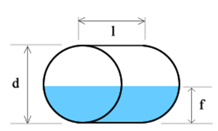To find the volume of a horizontal cylinder, we have to multiply its base area by its length. If the base is a circle of radius r, its area can be found as πr². Multiplying that by the length, we will get the total tank volume:

V = π × r² × l

Since r = d/2, the above formula can be re-written as follows:

V = π × r² × l = π × (d/2)² × l = (π × d² × l)/4

### Vertical cylinder tank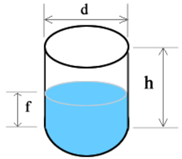The formula for the total volume of a vertical cylinder is the same as the formula for the horizontal cylinder, where the length, l, is replaced with the height, h:

V = π × r² × h = π × (d/2)² × h = (π × d² × h)/4

### Rectangular tank (rectangular prism)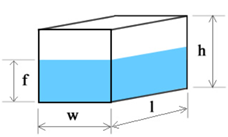This tank shape is widely known as "rectangular tank,"; however, this is not its official name. The rectangle is a 2D shape, and the tank is a rectangular prism. To find the volume of a rectangular prism, we have to multiply all three dimensions of the tank – width, length, and height:

V = w × l × h

### Horizontal oval tank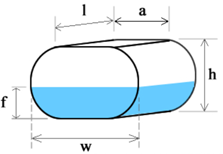This calculator defines an oval tank as a cylindrical tank with bases in a stadium shape. A stadium shape is defined as a rectangle with semicircles at opposite sides. To find the tank volume, we must multiply the base area by length.

Let's find the base area. The base area is represented by a stadium shape, as shown in the image below. The surface area of the stadium shape can be found by adding the rectangle surface area and the two semi-circles surface areas. Two semi-circles form one circle with radius r. Therefore, their combined area will be πr². The inner rectangle has sides with the following lengths: a and 2r. Its surface area can be found as 2ar.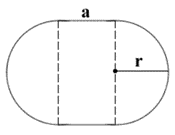The total surface area of the stadium shape can be found as πr² + 2ar.

The volume of a horizontal oval tank with the stadium shape base and length l can be found as follows:

V = (πr² + 2ar) × l

Since the calculator works in terms of the height of the cylinder, h, and h = 2r, the above formula can be re-written as follows:

r = h/2

V = (π(h/2)² + 2a(h/2)) × l = ((πh²)/4 + ah) × l

### Vertical oval tank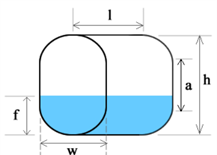While the volume of filled liquid for this tank will be different from the corresponding filled volume of the horizontal oval tank, the total volume formula is the same:

V = (πr² + 2ar) × l

In this case, w = 2r, and r = w/2, therefore, the formula can be re-written as follows:

V = (π(w/2)² + 2a(w/2)) × l = ((πw²)/4 + aw) × l

### Horizontal capsule tank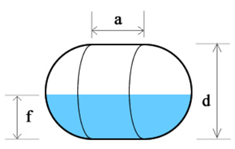This calculator defines a capsule as two hemispheres separated by a cylinder. Therefore, to find the volume of a capsule, we need to add the volume of the sphere to the volume of the inner cylinder. If the sphere has a radius r, its volume can be found as (4/3)πr³. The volume of a horizontal cylinder with base radius r and side length a can be found as πr2a. Therefore, the volume of the horizontal capsule tank can be found as follows:

V = (4/3)πr³ + πr²a = πr² × ((4/3)r + a)

Since r = d/2, the above formula can be re-written as follows:

V = π × (d/2)² × ((4d/6) + a)

### Vertical capsule tank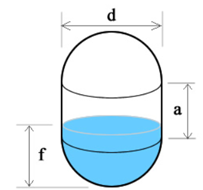While the volume of filled liquid for this tank will be different from the corresponding filled volume of the horizontal capsule tank, the total volume formula is the same:

V = πr² × ((4/3)r + a) = π × (d/2)² × ((4d/6) + a)

### Horizontal elliptical tank with 2:1 semi-elliptical tank heads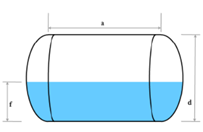This tank has semi-elliptical heads, with the ellipse's width being twice as long as its depth. If the straight length is a, then the depth of the head, let's denote it as H, will be a/4. Then the total volume of the tank heads can be calculated as follows:

Vₕ = πHd²/3

And the cylinder volume can be calculated as:

V꜀ = (π × d² × a)/4

The total tank volume will be:

V = Vₕ + V꜀

### Horizontal tank with dish ends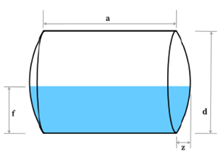The calculator on this page will also find a horizontal tank's total and filled volume with dish ends. However, the calculation formulas are extensive, and we will not show them here.

## Calculation example

An oil tank has a horizontal oval shape, a height of 3 meters, a width of 4 meters, and a length of 6 meters. The manual instructs that this tank cannot be filled above 90% of its total volume. What is the total volume of the tank? If you fill the tank up to the 2.5 meters depth, will you stay within the safe limits?

Let's use the calculator to find out the answers! First, choose "Horizontal Oval" from the drop-down menu. Then enter the known values:

h = 3 w = 4 l = 6 f = 2.5

After pressing "Calculate" we will see that the total tank volume is ≈ 60.4115 cubic meters or 15,959.03 U.S. gallons. We will also see that filling the tank up to the 2.5 meters depth will result in a fullness of 87.3%, which means you will stay within the safe limits.